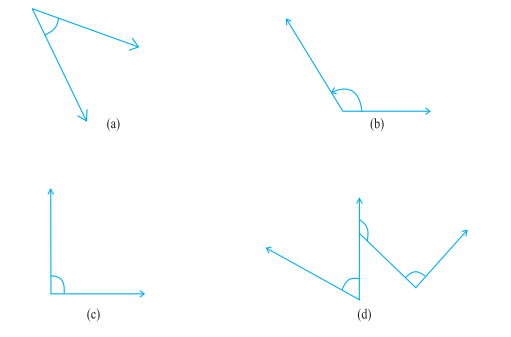## Filters

Sort by :
Clear All
Q

9. Find the angle measure between the hands of the clock in each figure :The angle measure between the hands of the clock in each figure is (a) 90o (b) 30o (c) 180o

8. Find the measure of the angle shown in each figure. (First estimate with your eyes and then find the actual measure with a protractor).(a) Measure of the given along = 40o  (b) Measure of the given along = 130o  (c) Measure of the given along = 65o  (d) Measure of the given along = 135o

7.  Fill in the blanks with acute, obtuse, right or straight :

(a) An angle whose measure is less than that of a right angle is______.

(b) An angle whose measure is greater than that of a right angle is ______.

(c) An angle whose measure is the sum of the measures of two right angles is _____.

(d) When the sum of the measures of two angles is that of a right angle, then each one of them is ______.

(e) When the sum of the measures of two angles is that of a straight angle and if one of them is acute then the other should be _______.

(a) An angle whose measure is less than that of a right angle is acute. (b) An angle whose measure is greater than that of a right angle is obtuse. (c) An angle whose measure is the sum of the measures of two right angles is straight. (d) When the sum of the measures of two angles is that of a right angle, then each one of them is acute. (e) When the sum of the measures of two angles is that of...

6.  From these two angles which has larger measure? Estimate and then confirm by measuring them.By estimation followed by confirmation by measurement we know that the second angle is greater.

5. Which angle has a large measure? First estimate and then measure.Measure of Angle A =

Measure of Angle B =

Measure of Angle A = 40o Measure of Angle B = 60o

4.Measure the angles given below using the Protractor and write down the measure.(a) 45o (b) 125o (c) 90o (d) 60o, 90o and 125o

3. Write down the measures of

(a) some acute angles.

(b) some obtuse angles.

(give at least two examples of each).

(a) 30o, 45o and 60o (b) 120o, 135o and 150o

2.  Say True or False :

(a) The measure of an acute angle < 90°.

(b) The measure of an obtuse angle < 90°.

(c) The measure of a reflex angle > 180°.

(d) The measure of one complete revolution = 360°.

(e) If m A∠ = 53° and m B∠ = 35°, then m A∠ > m B.

(a) True. (b) False. (c) True. (d) True. (e) True.

1. What is the measure of (i) a right angle? (ii) a straight angle?

(i) 90o (ii) 180o
Exams
Articles
Questions